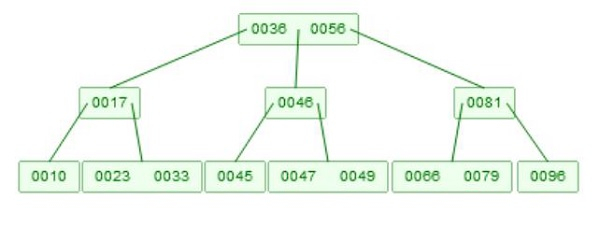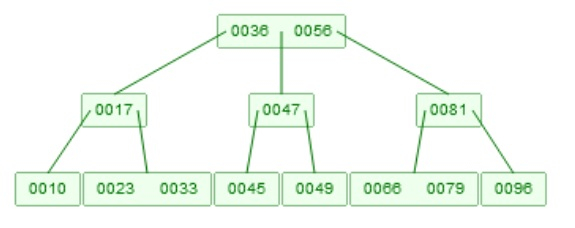# B-tree Deletion in Data Structure

Here we will see, how to perform the deletion of a node from B-Tree. Suppose we have a BTree like below −

Example of B-TreeDeletion has two parts. At first we have to find the element. That strategy is like the querying. Now for deletion, we have to care about some rules. One node must have at-least m/2 elements. So if we delete, one element, and it has less than m-1 elements remaining, then it will adjust itself. If the entire node is deleted, then its children will be merged, and if their size issame as m, then split them into two parts, and again the median value will go up.

Suppose we want to delete 46. Now there are two children. , and [47, 49], then they will be merged, it will be [45, 47, 49], now 47 will go up.## Algorithm

BTreeDelete(x, key) −

Input − The root of the tree, and key to delete

We will assume, that the key is present into the list

if x is leaf, then
delete object with key ‘key’ from x
else if x does not contain the object with key ‘key’, then
locate the child x->child[i] whose key range is holding ‘key’
y := x->child[i]
if y has m/2 elements, then
If the sibling node z immediate to the left or right of y, has at least one more
object than m/2, add one more object by moving x->key[i] from x to y, and
move that last or first object from z to x. If y is non-leaf node, then last or first
child pointer in z is also moved to y
else
any immediate sibling of y has m/2 elements, merge y with immediate sibling
end if
BTreeDelete(y, key)
else
if y that precedes ‘key’ in x, has at-least m/2 + 1 objects, then
find predecessor k of ‘key’, in the sub-tree rooted by y. then recursively delete k
from the sub-tree and replace key with k in x
else if ys has m/2 elements, then
check the child z, which is immediately follows ‘key’ in x
if z has at least m/2+1 elements, then
find successor k of ‘key’, in the sub-tree rooted by z. recursively delete k
from sub-tree, and replace key with k in x
else
both y and z has m/2 elements, then merge then into one node, and push ‘key’
down to the new node as well. Recursively delete ‘key’ from this new node
end if
end if